# Top 83 CAT Functions, Graphs and Statistics Questions With Solutions

CAT Functions, Graphs and Statistics questions are very important to practice while preparing for the CAT exam. Every year these questions frequently appear in the CAT question papers. Check out the below-given practice questions from this topic(Functions, Graphs and Statistics). All these questions are taken from CAT's previous papers and separated year-wise. One can practice these questions in a test format or can also download these questions in a PDF format along with the detailed video solutions for every question explained by the CAT toppers. Click on the below link to download the CAT Functions, Graphs and Statistics Questions PDF.

## CAT Functions, Graphs and Statistics Questions Weightage Over Past 5 Years

 Year Weightage 2022 5 2021 1 2020 8 2019 5 2018 3

## CAT 2022 Functions, Graphs and Statistics questions

#### Question 1

Let $$0 \leq a \leq x \leq 100$$ and $$f(x) = \mid x - a \mid + \mid x - 100 \mid + \mid x - a - 50\mid$$. Then the maximum value of f(x) becomes 100 when a is equal to

#### Question 2

Let $$f(x)$$ be a quadratic polynomial in $$x$$ such that $$f(x) \geq 0$$ for all real numbers $$x$$. If f(2) = 0 and f( 4) = 6, then f(-2) is equal to

#### Question 3

Suppose for all integers x, there are two functions f and g such that $$f(x) + f (x - 1) - 1 = 0$$ and $$g(x ) = x^{2}$$. If $$f\left(x^{2} - x \right) = 5$$, then the value of the sum f(g(5)) + g(f(5)) is

#### Question 4

Let r be a real number and $$f(x) = \begin{cases}2x -r & ifx \geq r\\ r &ifx < r\end{cases}$$. Then, the equation $$f(x) = f(f(x))$$ holds for all real values of $$x$$ where

#### Question 5

For any real number x, let [x] be the largest integer less than or equal to x. If $$\sum_{n=1}^N \left[\frac{1}{5} + \frac{n}{25}\right] = 25$$ then N is

## CAT 2021 Functions, Graphs and Statistics questions

#### Question 1

If $$f(x)=x^{2}-7x$$ and $$g(x)=x+3$$, then the minimum value of $$f(g(x))-3x$$ is:

## CAT 2020 Functions, Graphs and Statistics questions

#### Question 1

Let $$f(x)=x^{2}+ax+b$$ and $$g(x)=f(x+1)-f(x-1)$$. If $$f(x)\geq0$$ for all real x, and $$g(20)=72$$. then the smallest possible value of b is

#### Question 2

The number of real-valued solutions of the equation $$2^{x}+2^{-x}=2-(x-2)^{2}$$ is:

#### Question 3

The area of the region satisfying the inequalities $$\mid x\mid-y\leq1,y\geq0$$ and $$y\leq1$$ is

#### Question 4

In a group of 10 students, the mean of the lowest 9 scores is 42 while the mean of the highest 9 scores is 47. For the entire group of 10 students, the maximum possible mean exceeds the minimum possible mean by

#### Question 5

The area, in sq. units, enclosed by the lines $$x=2,y=\mid x-2\mid+4$$, the X-axis and the Y-axis is equal to

#### Question 6

If $$f(x+y)=f(x)f(y)$$ and $$f(5)=4$$, then $$f(10)-f(-10)$$ is equal to

#### Question 7

If $$f(5+x)=f(5-x)$$ for every real x, and $$f(x)=0$$ has four distinct real roots, then the sum of these roots is

#### Question 8

In how many ways can a pair of integers (x , a) be chosen such that $$x^{2}-2\mid x\mid+\mid a-2\mid=0$$ ?

## CAT 2019 Functions, Graphs and Statistics questions

#### Question 1

Consider a function f satisfying f (x + y) = f (x) f (y) where x,y are positive integers, and f(1) = 2. If f(a + 1) +f (a + 2) + ... + f(a + n) = 16 (2$$^n$$ - 1) then a is equal to

#### Question 2

For any positive integer n, let f(n) = n(n + 1) if n is even, and f(n) = n + 3 if n is odd. If m is a positive integer such that 8f(m + 1) - f(m) = 2, then m equals

#### Question 3

The number of the real roots of the equation $$2 \cos (x(x + 1)) = 2^x + 2^{-x}$$ is

#### Question 4

Let S be the set of all points (x, y) in the x-y plane such that $$\mid x \mid + \mid y \mid \leq 2$$ and $$\mid x \mid \geq 1.$$ Then, the area, in square units, of the region represented by S equals

#### Question 5

Let f be a function such that f (mn) = f (m) f (n) for every positive integers m and n. If f (1), f (2) and f (3) are positive integers, f (1) < f (2), and f (24) = 54, then f (18) equals

## CAT 2018 Functions, Graphs and Statistics questions

#### Question 1

Let f(x)= $$\max(5x, 52-2x^2)$$, where x is any positive real number. Then the minimum possible value of f(x)

#### Question 2

Let f(x) = min ($${2x^{2},52-5x}$$) where x is any positive real number. Then the maximum possible value of f(x) is

#### Question 3

If $$f(x + 2) = f(x) + f(x + 1)$$ for all positive integers x, and $$f(11) = 91, f(15) = 617$$, then $$f(10)$$ equals

## CAT 2017 Functions, Graphs and Statistics questions

#### Question 1

Let $$f(x) = x^{2}$$ and $$g(x) = 2^{x}$$, for all real x. Then the value of f[f(g(x)) + g(f(x))] at x = 1 is

#### Question 2

If $$f_{1}(x)=x^{2}+11x+n$$ and $$f_{2}(x)=x$$, then the largest positive integer n for which the equation $$f_{1}(x)=f_{2}(x)$$ has two distinct real roots is

#### Question 3

If f(ab) = f(a)f(b) for all positive integers a and b, then the largest possible value of f(1) is

#### Question 4

Let $$f(x) =2x-5$$ and $$g(x) =7-2x$$. Then |f(x)+ g(x)| = |f(x)|+ |g(x)| if and only if

#### Question 5

$$f(x) = \frac{5x+2}{3x-5}$$ and $$g(x) = x^2 - 2x - 1$$, then the value of $$g(f(f(3)))$$ is

## CAT 2008 Functions, Graphs and Statistics questions

#### Question 1

Let $$f(x)\neq0$$ for any 'x' be a function satisfying $$f(x)f(y) = f(xy)$$ for all real x, y. If $$f(2) = 4$$, then what is the value of $$f(\frac{1}{2})$$?

#### Question 2

Suppose, the seed of any positive integer n is defined as follows:

seed(n) = n, if n < 10

seed(n) = seed(s(n)), otherwise, where s(n) indicates the sum of digits of n.

For example, seed(7) = 7,

seed(248) = seed(2 + 4 + 8) = seed(14) = seed (1 + 4) = seed (5) = 5 etc.

How many positive integers n, such that n < 500, will have seed (n) = 9?

#### Question 3

Find the sum $$\sqrt{1+\frac{1}{1^2}+\frac{1}{2^2}}+\sqrt{1+\frac{1}{2^2}+\frac{1}{3^2}} +....+ \sqrt{1+\frac{1}{2007^2}+\frac{1}{2008^2}}$$

#### Question 4

Let $$f(x) = ax^2 + bx + c$$, where a, b and c are certain constants and $$a \neq 0$$ ?

It is known that $$f(5) = - 3f(2)$$. and that 3 is a root of $$f(x) = 0$$.

What is the other root of f(x) = 0?

[CAT 2008]

#### Question 5

Let $$f(x) = ax^2 + bx + c$$, where a, b and c are certain constants and $$a \neq 0$$ ?

It is known that f(5) = - 3f(2). and that 3 is a root of f(x) = 0.

What is the value of a + b + c?

[CAT 2008]

## CAT 2007 Functions, Graphs and Statistics questions

#### Question 1

A function $$f (x)$$ satisfies $$f(1) = 3600$$, and $$f (1) + f(2) + ... + f(n) =n^2f(n)$$, for all positive integers $$n > 1$$. What is the value of $$f (9)$$ ?

#### Question 2

Which of the following best describes $$a_n + b_n$$ for even n?

#### Question 3

If p = 1/3 and q = 2/3 , then what is the smallest odd n such that $$a_n+b_n < 0.01$$?

#### Question 4

For general n, consider any two members of S that are friends. How many other members of S will be common friends of both these members?

#### Question 5

For general n, how many enemies will each member of S have?

## CAT 2006 Functions, Graphs and Statistics questions

#### Question 1

The graph of y - x (on the y axis) against y + x (on the x axis) is as shown below. (All graphs in this question are drawn to scale and the same scale and the same scale has been used on each axis.)

Which of the following shows the graph of y against x?

#### Question 2

Let f(x) = max (2x + 1, 3 - 4x), where x is any real number. Then the minimum possible value of f(x) is:

## CAT 2005 Functions, Graphs and Statistics questions

#### Question 1

If $$a_1 = 1$$ and $$a_{n+1} - 3a_n + 2 = 4n$$ for every positive integer n, then $$a_{100}$$ equals

#### Question 2

In the X-Y plane, the area of the region bounded by the graph of |x+y| + |x-y| = 4 is

#### Question 3

Let g(x) be a function such that g(x+1) + g(x-1) = g(x) for every real x. Then for what value of p is the relation g(x+p) = g(x) necessarily true for every real x?

## CAT 2004 Functions, Graphs and Statistics questions

#### Question 1

If $$f(x)=x^3-4x+p$$ , and f(0) and f(1) are of opposite signs, then which of the following is necessarily true

[CAT 2004]

#### Question 2

Let $$y = \frac{1}{2+\frac{1}{3+\frac{1}{2+\frac{1}{3+…}}}}$$. Then y equals?

#### Question 3

Let $$f(x) = ax^2 - b|x|$$ , where a and b are constants. Then at x = 0, f(x) is

[CAT 2004]

#### Question 4

If $$\frac{a}{b+c}=\frac{b}{a+c} =\frac{c}{b+a} =r$$, then r cannot take any value except

#### Question 5

How many of the following products are necessarily zero for every x:

$$f_1(x)f_2(x), f_2(x)f_3(x), f_2(x)f_4(x)$$

#### Question 6

Which of the following is necessarily true?

## CAT 2003 Functions, Graphs and Statistics questions

#### Question 1

When the curves $$y = log_{10}x$$ and $$y = x^{-1}$$ are drawn in the x-y plane, how many times do they intersect for values $$x \geq 1$$ ?

#### Question 2

Let g(x) = max(5 - x, x + 2). The smallest possible value of g(x) is

#### Question 3

The function f(x) = |x - 2| + |2.5 - x| + |3.6 - x|, where x is a real number, attains a minimum at

#### Question 4

Consider the following two curves in the x-y plane:

$$y = x^3 + x^2 + 5$$

$$y = x^2 + x + 5$$

Which of following statements is true for $$-2 \leq x \leq 2$$ ?

## CAT 2002 Functions, Graphs and Statistics questions

#### Question 1

Suppose for any real number x, [x] denotes the greatest integer less than or equal to x. Let L(x, y) = [x] + [y] + [x + y] and R(x, y) = [2x] + [2y]. Then it is impossible to find any two positive real numbers x and y for which

## CAT 2000 Functions, Graphs and Statistics questions

#### Question 1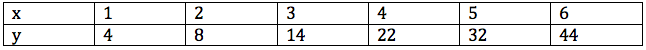In the above table, for suitably chosen constants a, b and c, which one of the following best describes the relation between y and x?

#### Question 2

The area bounded by the three curves |x+y| = 1, |x| = 1, and |y| = 1, is equal to:

#### Question 3

The set of all positive integers is the union of two disjoint subsets:

{f(1), f(2),.....f(n), ...} and {g(1),g(2).... ,g(n).....}, where f(1) < f(2) <.....< f(n)..., and g(1) < g(2) < ..... < g(n) ...,and

g(n) = f(f(n))+1 for all n >= 1. What is the value of g(1)?

#### Question 4

For all non-negative integers x and y, f(x, y) is defined as below:

f(0, y) = y + 1

f(x + 1, 0) = f(x, 1)

f(x+ 1, y+ 1)= f(x, f(x+ 1, y))

Then, what is the value of f(1,2)?

#### Question 5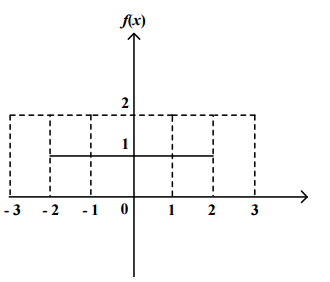#### Question 6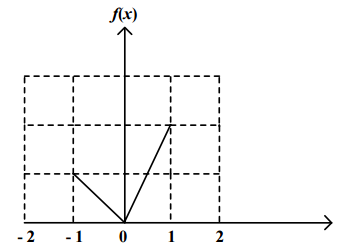#### Question 8

What is the value of the product, $$f(2) f^2(2)f^3(2) f^4(2)f^5(2)$$?

#### Question 9

r is an integer 2. Then, what is the value of $$f^{r-1}(-r) + f^r(-r) + f^{r+1}(-r)$$?

#### Question 10

Which of the following is necessarily greater than 1?

#### Question 11

Which of the following expressions is necessarily equal to 1?

#### Question 12

Which of the following expressions is indeterminate?

#### Question 13

Which of the following expressions yields a positive value for every pair of non-zero real numbers (x, y)?

#### Question 14

Under which of the following conditions is f(x, y) necessarily greater than g(x, y)?

## CAT 1999 Functions, Graphs and Statistics questions

#### Question 1

For two positive integers a and b define the function h(a,b):as the greatest common factor (G.C.F) of a, b. Let A be a set of n positive integers. G(A), the GCF of the elements of set A is computed by repeatedly using the function h.
The minimum number of times h is required to be used to compute G is:

#### Question 2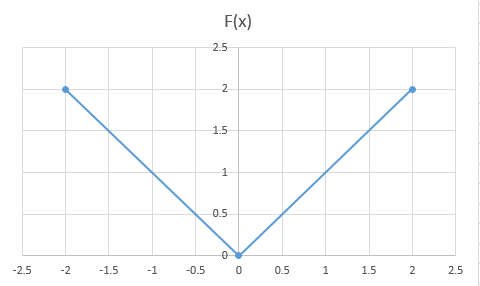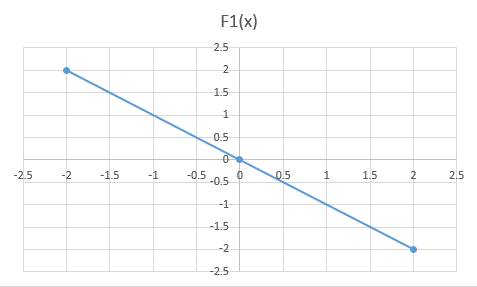#### Question 3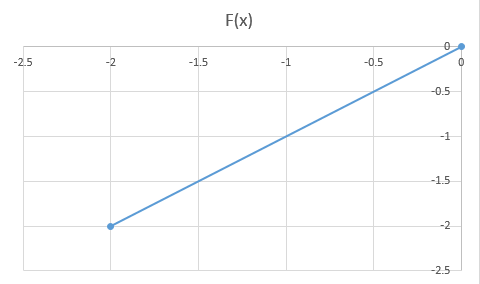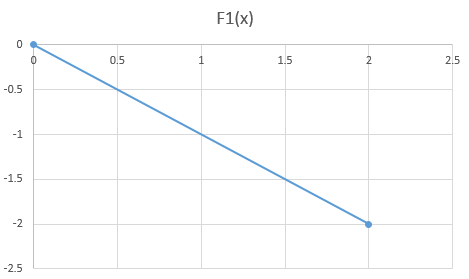#### Question 4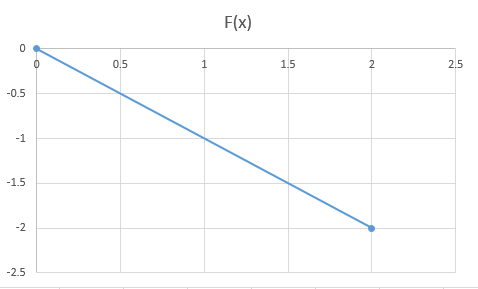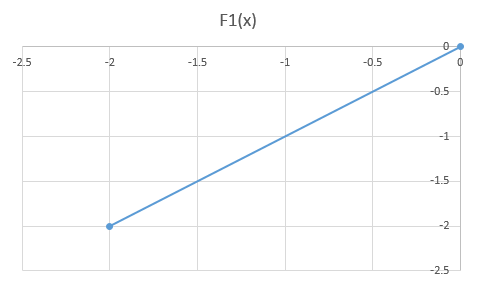#### Question 5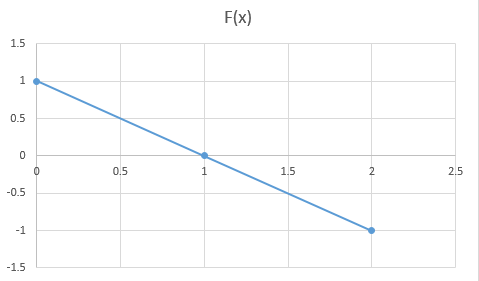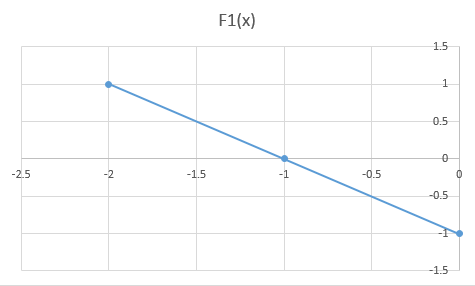#### Question 6

Which of the following statements is true?

#### Question 7

What is the value of f(G(f(1, 0)), f(F(f(1, 2)), G(f(1, 2))))?

#### Question 8

Which of the following expressions yields $$x^2$$ as its result?

#### Question 9

The robot reaches point (6, 6) when a sequence of three instructions is executed, the first of which is a GOTO(x, y) instruction, the second is WALKX(2) and the third is WALKY(4). What are the values of x and y?

#### Question 10

The robot is initially at (x, y), x > 0 and y < 0. The minimum number of instructions needed to be executed to bring it to the origin (0,0) if you are prohibited from using the GOTO instruction is:

## CAT 1996 Functions, Graphs and Statistics questions

#### Question 1

What is the value of $$M(M(A(M(x, y),S(y, x)),x),A(y, x))$$for $$x=2, y=3$$?

#### Question 2

What is the value of $$S[M(D(A(a, b), 2), D(A(a, b), 2)), M(D(S(a, b), 2), D(S(a, b), 2))]$$?

## CAT 1991 Functions, Graphs and Statistics questions

#### Question 1

A function can sometimes reflect on itself, i.e. if y = f(x), then x = f(y). Both of them retain the same structure and form. Which of the following functions has this property?

#### Question 2

What is the value of k for which the following system of equations has no solution:
2x-8y = 3 and kx +4y = 10

#### Question 3

If $$y = f(x)$$ and $$f(x) = \frac{(1-x)}{(1 + x)}$$, which of the following is true?

#### Question 4

Let Y = minimum of {(x+2), (3-x)}. What is the maximum value of Y for 0 <= x <=1?